## Parabolic Segment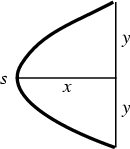The Arc Length of the parabolic segment shown above is given by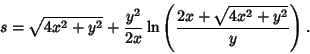(1)

The Area contained between the curves(2)(3)

can be found by eliminating,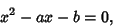(4)

so the points of intersection are(5)

Therefore, for the Area to be Nonnegative,, and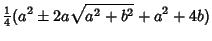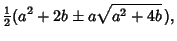(6)

so the Area is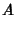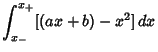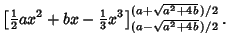(7)

Now,(8)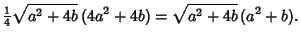(9)

So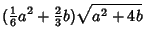(10)

We now wish to find the maximum Area of an inscribed Triangle. This Triangle will have two of its Vertices at the intersections, and Area(11)

But, so(12)

The maximum Area will occur when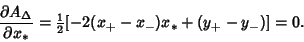(13)

But(14)(15)

so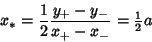(16)

and(17)

Working on the third term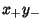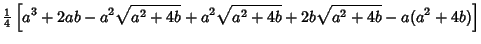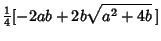(18)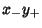(19)

so(20)

and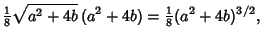(21)

which gives the result known to Archimedesin the third century BCthat(22)

The Area of the parabolic segment of height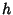opening upward along the y-Axis is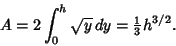(23)

The weighted mean ofis(24)

The Centroid is then given by(25)